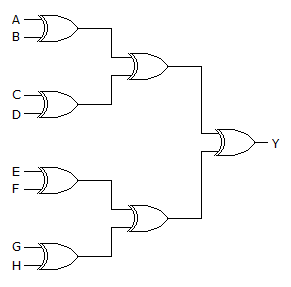# Pandora's Box

25 Questions | Total Attempts: 199SettingsInstructions:Questions are based on basics of C/C++ , digital and analog electronics. There are no negative marks For descriptive type of questions, try to answer precisely. Submit the answers before time runs. ​ALL THE BEST!

Related Topics
• 1.
The terminal count of a modulus-25 binary counter is
• A.

11001

• B.

11000

• C.

10111

• D.

100000

• 2.
A ring oscillator consisting of 4 inverters is running at a frequency of 1MHz . The propagation delay per gate is
• A.

1.25 nsec

• B.

125nsec

• C.

0.1nsec

• D.

10ns

• 3.
Let k=2n.  A circuit is built by giving the output of an n-bit binary counter as input to an n to 2n bit decoder. This circuit is equivalent to a
• A.

K-bit binary up counter

• B.

K- bit ring counter

• C.

k-bit binary down counter

• D.

K-bit Johnson counter

• 4.
Assume that a 5 bit serial in/serial out shift register is initially has 10101 . If the data to be sent is 11000 (starting with the left most bit). What would be the 5-bit-pattern after fourth clock pulse.
• A.

10111

• B.

01110

• C.

11100

• D.

11000

• 5.
Consider the following:a. pointer to a function that accepts 3 integer arguments and returns a floating-point quantityfloat(*pf)(int a,int b,int c);b. Pointer to a function that accepts 3 pointers to integer quantities as arguments and returns a pointer to a floating point quantityfloat*(*pf)(int*a,int*b,int*c);
• A.

A is true, b is true

• B.

A is true, b is false

• C.

A is false, b is false

• D.

A is false, b is true

• 6.
A mod2 counter followed by the mod5 counter is
• A.

Same as mod5 counter

• B.

Same mod2 counter

• C.

Mod7 counter

• D.

• 7.
Main(){ char*x=”String”; char y[]=”add”; char *z; z= (char *)malloc(sizeof(x)+sizeof(y)=1);strcpy(z,y);strcat(z,y);printf(“%s+%s=%s”,y,x,z);}
• A.

• B.

Runtimeerror/coredump

• C.

Syntax error during compilation

• D.

• 8.
Main(void){void f(int,int);int i=10;f(i,i++);}Void f(int i,int j){   if(i>50)    return;  i+=j;f(i,j);printf(“%d”,i);}
• A.

85,53,32,21

• B.

10,11,21,32,53

• C.

32,21,11,10

• D.

None of the above

• 9.
Main(void){ int i=9;Printf(“%old  %old %old %old”,i,i++,i--,i++);}
• A.

8ld 8ld 9ld 8ld

• B.

9ld 8ld 9ld 8ld

• C.

10ld 10ld 11ld 10ld

• D.

11ld 10ld 11ld 10ld

• 10.
Suppose the time taken for executing a c program( containing 1000 lines code )is 1msec and if we add 100 comments (each comment contains 10 characters )in between then the execution time will be
• A.

Double

• B.

Same

• C.

Four times

• D.

Data not sufficient to determine

• 11.
Determine the forward resistance of a pn junction diode when the forward current is 5mA at T=300k (assume silicon diode)
• A.

9.3 ohms

• B.

10.20 ohms

• C.

10.34 ohms

• D.

10.50 ohms

• 12.
Difference between resistor and inductor?
• 13.
Kirchhoff’s laws are valid for
• A.

Linear circuit only

• B.

Passive time invariant circuits

• C.

Non-linear circuit’s only

• D.

Both linear and non-linear circuits.

• 14.
Why are most interrupts active low?
• 15.
What is virtual grounding?
• 16.
Removing bypass capacitor across the emitter-leg resistor in a CE amplifier causes
• A.

Increase in current gain

• B.

Decrease in current gain

• C.

Increase in voltage gain

• D.

Decrease in voltage gain

• 17.
What are the minimum number of 2 to 1 multiplexers required to generate a 2-input AND gate and a 2-input EX-OR gate?
• A.

1 and 2

• B.

1 and 3

• C.

1 and 1

• D.

2 and 2

• 18.
How the op-amp comparator should be chosen to get higher speed of operation?
• A.

Large gain

• B.

High slew rate

• C.

Wider bandwidth

• D.

None of the mentioned

• 19.
The 8-input XOR circuit shown has an output of Y = 1. Which input combination below (ordered A – H) is correct?
• A.

10111100

• B.

10111000

• C.

11100111

• D.

00011101

• 20.
Convert the following SOP expression to an equivalent POS expression
• A.
• B.
• C.
• D.
• 21.
What is X?
• A.

2-input AND gate

• B.

2-input OR gate

• C.

Exclusive-OR gate

• D.

None of the above

• 22.
Find collector current IC2, given input voltages are V1=2.078v & V2=2.06v and total current IQ=2.4mA. (Assume α=1)
• A.

0.8mA

• B.

1.6mA

• C.

0.08mA

• D.

0.16mA

• 23.
The binary code of (21.125)10 is
• A.

10101.001

• B.

10100.001

• C.

10101.010

• D.

None of the above

• 24.
A debouncing circuit is
• A.

An astable multi vibrator

• B.

A bistable multivibrator

• C.

A latch

• D.

Xor gate

• 25.
On the third clock pulse, a 4-bit Johnson sequence is Q0 = 1, Q1 = 1, Q2 = 1, and Q3 = 0. On the fourth clock pulse, the sequence is.....
• A.

Q0 = 1, Q1 = 1, Q2 = 1, Q3 = 1

• B.

Q0 = 1, Q1 = 1, Q2 = 0, Q3 = 0

• C.

Q0 = 1, Q1 = 0, Q2 = 0, Q3 = 0

• D.

Q0 = 0, Q1 = 0, Q2 = 0, Q3 = 0